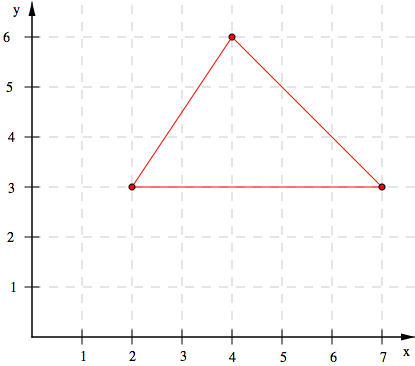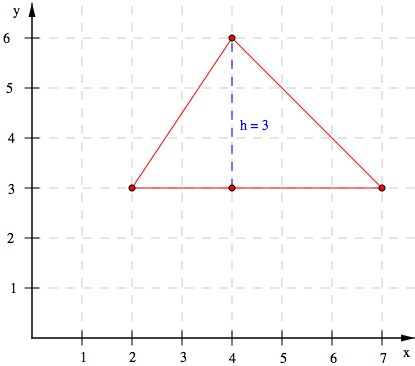### Home > MC1 > Chapter 10 > Lesson 10.2.3 > Problem10-104

10-104.

On a coordinate grid, plot the points $\left(2,3\right)$, $\left(4,6\right)$, and $\left(7,3\right)$.

1. Connect the points to form a triangle.

2. Find the area of this triangle.

$\text{Area }= \frac{1}{2}bh$